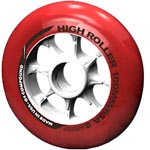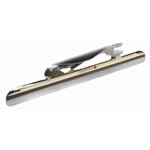Resistance of friction in skating (roller or ice)

For a skater, the friction (non-aerodynamic) comes from the contact of the skates on the ground.

The contact requires a sufficient grip to prevent falls and provide thrusts but generates in return a resistance Rf. This resistance is proportionate to the total weight through a coefficient Cf and depends on the slope Pt (slope calculation). The total weight depends on the total mass Mt (skater plus equipment) and the acceleration of gravity g.

Contact of the wheels (roller)

With wheels, the resistance Rf is named rolling resistance Rr.

The associated coefficient Cr defines the rolling efficiency of wheels. It depends on the nature of the ground (asphalt, cement, etc.) and the type of tread (constitution, form, size).

 For good skates (polyurethane wheels), we can consider: Cr = 0.014 for a bad asphalt road Cr = 0.012 for a good asphalt road Cr = 0.010 for a very smooth asphalt road Cr = 0.008 for a velodrome trackN.B. There is an additional resistance named friction resistance (friction in bearings, etc.), but it is totally negligible beside the previous resistance.

With blades, the resistance Rf is named sliding resistance Rg.

The associated coefficient Cg defines the sliding efficiency of blades. It depends on the nature of the ice (quality, etc.) and the type of blades (constitution, form, size).

 For good skates, we can consider: Cg = 0.006 for a bad ice Cg = 0.005 for a good ice Cg = 0.004 for a very smooth iceCalculation formula

In summary, the mathematic formula allowing calculation is:
Rf = Mt g Cf Cos(Arctan(Pt))

Calculation of the skating power

J.L